MORE IN Design and Analysis of Algorithms
VTU Computer Science (Semester 4)
Design and Analysis of Algorithms
December 2012
Total marks: --
Total time: --
INSTRUCTIONS
(1) Assume appropriate data and state your reasons
(2) Marks are given to the right of every question
(3) Draw neat diagrams wherever necessary

1 (a) Define asymptotic notations.
3 M
1 (b) Algorithm X(int N)
{
{
int P;
for i? 1 to N
{
printf(" %d \t * \t %d=%d", N, i, P);
P-P+N;
}
}
i) What does this algorithm compute?
ii) What is the basic operation?

iii) How many times the basic operation is executed?
iv) What is the efficiency class of this algorithm?
4 M
1 (c) Solve the following recurrence relation $\ f(n)= \left\{\begin{matrix} f(n-1) &n> 0 \\ 0 & n=0 \end{matrix}\right.$ $\begin{matrix}x(n)=3x(n-1) &for \ n>1, \ x(1)=4 \\x(n) =x(n|2)+n &for \ n>1,\ x(1) =1 n=2^k\end{matrix}$
4 M
1 (d) Sort the list EXAMPLE by dubbed sort, ls there any possibilities that bubble sort can be stopped earlier?
5 M

2 (a) Discuss how quick sort work to sort an array. Trace quick sort algorithm for the following data set 65, 70, 75, 80, 85, 60, 55, 50, 45. Also derive the worst case complexity of quick sort.
9 M
2 (b) Write the recursive algorithm for merge sort.
4 M
2 (c) Consider the following set of 14 elements in an array list, -15, -6, 0, 7, 9, 23, 54, 82, 101, 112, 125, 131, 142, 151 when binary search is applied on these elements, find the elements which required maximum number of comparisons. Also determine average number of key comparison for successful search and unsuccessful search.
4 M
2 (d) Derive the time complexity for defective chess board.
3 M

3 (a) Solve the following instance of knapsack problem, using greedy algorithm
 Item 1 2 3 4 Weight 4 7 5 3 Profit 40 42 25 12

Knapsack weight M=10
5 M
3 (b) Using Prim's algorithm, determine minimum cost spanning tree for the following graph Fig Q3(b)
How Knapsack and Prim's algorithms guarantee the elimination of cycles?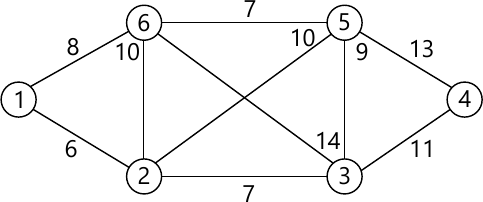7 M
3 (c) In the above graph fig. Q3(c), determine the shortest distances from source vertex 5 to all the remaining vertices, using Dijkstra's algorithm.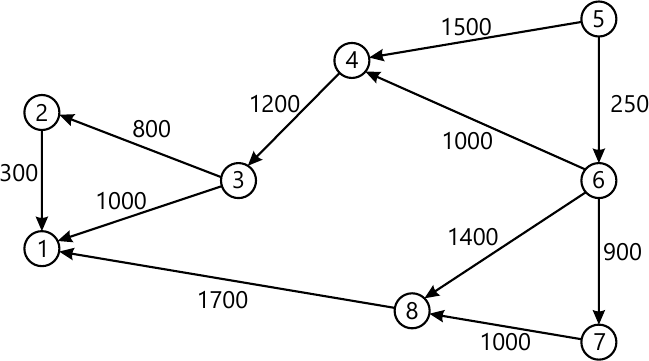8 M

4 (a) Solve the following travelling sales person problem, using dynamic programming
$\begin{bmatrix} 0 &10 &15 &20 \\5 &0 &9 &10 \\6 &13 &0 &12 \\8 &8 &9 &0 \end{bmatrix}$
Starting city 1
10 M
4 (b) Write Warshall - Floyd algorithm
3 M
4 (c) Generate the transitive closure of the graph is given below.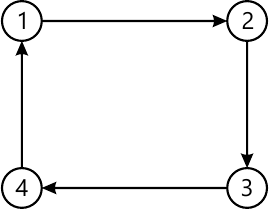7 M

5 (a) Match the pattern BAOBAB in the text BESS-KNEW-ABOUT-BAOBAS, using
i) Horspool's algorithm
ii) Boyer Moore algorithm
8 M
5 (b) Write a BFS algorithm to check the connectivity of a given graph.
5 M
5 (c) Apply source elimination based algorithm to represent vertices in topological ordering for the digraph given in Fig Q5(c).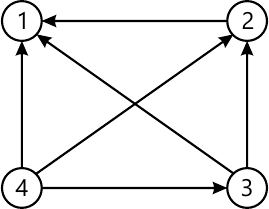4 M
5 (d) Apply distribution counting algorithm to sort the elements b, c, d, c, b, a, a, b.
3 M

6 (a) What are decision trees? Explain with example, how decision trees are used in sorting algorithms.
10 M
6 (b) Explain the concepts of P, NP and NP - complete problems.
10 M

7 (a) Draw the state-space tree to generate solutions to 4-Queen's problem.
4 M
7 (b) Apply back tracking method to solve subset sun problem for the instance n=6, d=30. S={5, 10, 12, 13, 15, 18}
6 M
7 (c) What is branch and bound algorithm? How it is different from backtracking?
5 M
7 (d) Write the steps and apply nearest neighbour approximation algorithm on the TSP problem with the starting vertex a, and calculate the accuracy ratio of approximation.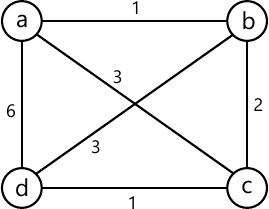5 M

8 (a) What are the different computation models? Discuss in detail.
10 M
8 (b) Let the input to the prefix computation problem be 5, 12, 8, 6, 3, 9, 11, 12, 5, 6, 7, 10, 4, 3, 5 and let ? stand for addition. Solve the problem using work optimal algorithm.
10 M

More question papers from Design and Analysis of Algorithms